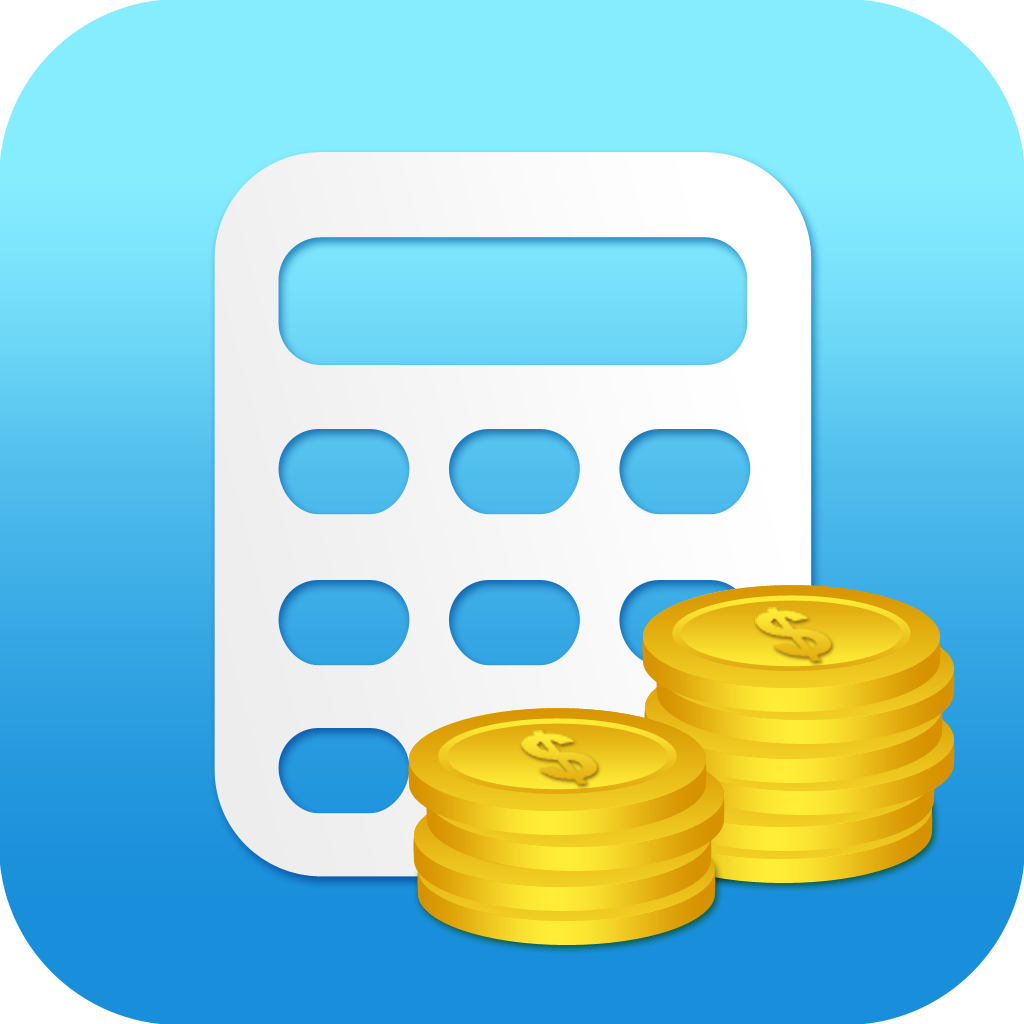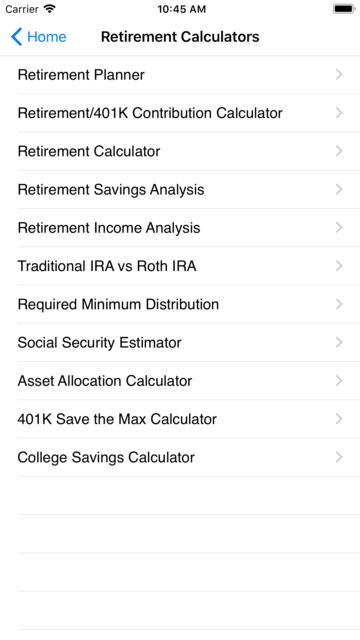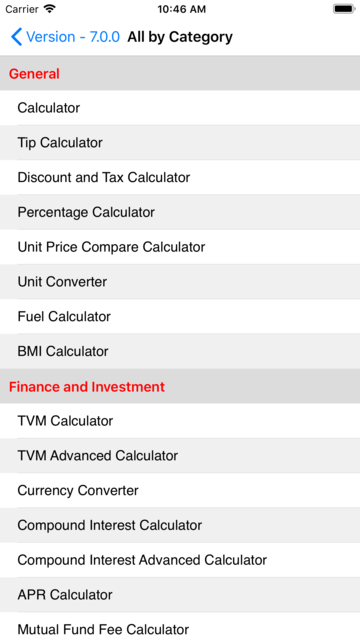### Description

For phone and tablet, this application includes the complete package of financial calculators by Bishinew Inc:

Finance and Investment Calculators

* TVM Calculator
* Currency Converter
* Compound Interest Calculator
* APR Calculator
* Mutual Fund Fee Calculator
* Return On Investment (ROI) Calculator
* Rule of 72 Calculator
* Effective Rate Calculator
* IRR NPV Calculator
* MIRR Calculator
* Tax Equivalent Yield Calculator
* US Inflation Calculator
* Salary Increase Calculator
* US Paycheck Tax Calculator
* Net Distribution Tax Calculator
* Health Savings Account Calculator

Loan/Mortgage Calculators

* Loan Calculator
* Loan Comparison Calculator
* Loan Refinance Calculator
* Loan Analysis Calculator
* Commercial Loan Calculator
* Loan Term Calculator
* Fixed Principal Loan Calculator
* Home Affordability Loan Calculator
* Rent vs Buy Loan Calculator
* Mortgage Tax Saving Loan Calculator
* Discount Points Loan Calculator
* Fixed vs Adjustable Rate Loan Calculator
* Bi-weekly Payment Loan Calculator
* Interest Only Loan Calculator
* Rule of 78 Loan Calculator

Retirement Calculators

* Retirement/401k Contribution Calculator
* Retirement Calculator
* Retirement Savings Analysis
* Retirement Income Analysis
* Traditional IRA vs Roth IRA - Retirement
* Required Minimum Distribution - Retirement
* Social Security Estimator - Retirement
* Asset Allocation Calculator - Retirement
* Start Early and Save Regularly - Retirement
* Set Goal for Retirement - Retirement
* Max Out 401k/IRA Savings - Retirement
* Invest Wisely - Retirement
* How Much Money Will I Need? - Retirement
* How Long Does My Money Last? - Retirement
* How Much Should I Withdraw? - Retirement
* Inflation Matters - Retirement
* 401k Save the Max Calculator
* College Savings Calculator

Bond Calculators

* Bond Calculator
* Bond Yield to Call (YTC) Calculator
* Bond Yield to Maturity (YTM) Calculator
* Bond Duration Calculator

Stock Calculators

* Stock Return Calculator
* Stock Constant Growth Calculator
* Stock Non-constant Growth Calculator
* CAPM Calculator
* Expected Return Calculator
* Holding Period Return Calculator
* Weighted Average Cost of Capital Calculator
* Pivot Point Calculator
* Fibonacci Calculator
* Black-Scholes Option Calculator

Credit Card Calculators

* Credit Card Payoff Calculator
* Credit Card Minimum Calculator

Auto Loan and Lease Calculators

* Auto Loan Calculator
* Auto Lease Calculator

* Depreciation Calculator
* Break Even Point Calculator
* Margin and Markup Calculator
* Financial Ratios

General Calculators

* Regular Calculator
* Tip Calculator
* Discount and Tax Calculator
* Percentage Calculator
* Unit Price Compare Calculator
* Unit Conversion
* Date Calculator
* Fuel Calculator
* BMI Calculator

User can send the calculation results to others via Email. Financial Professionals can email the quote to their clients.

The app allows you to edit and prioritize the list of calculators for easy access.

Only Currency Converter needs internet access to retrieve the latest currency exchange rate. The app works without internet connection.

If you have any question or report issue, please send email to pfinanceapp@gmail.com. We will reply right away.

Primary: Finance

### Total Ratings

360
 5 250 4 27 3 23 2 20 1 47

### Screenshots### Version History

Launched Jan 17, 2012 (about 9 years ago).

Releasing new versions every 5 months, on average.